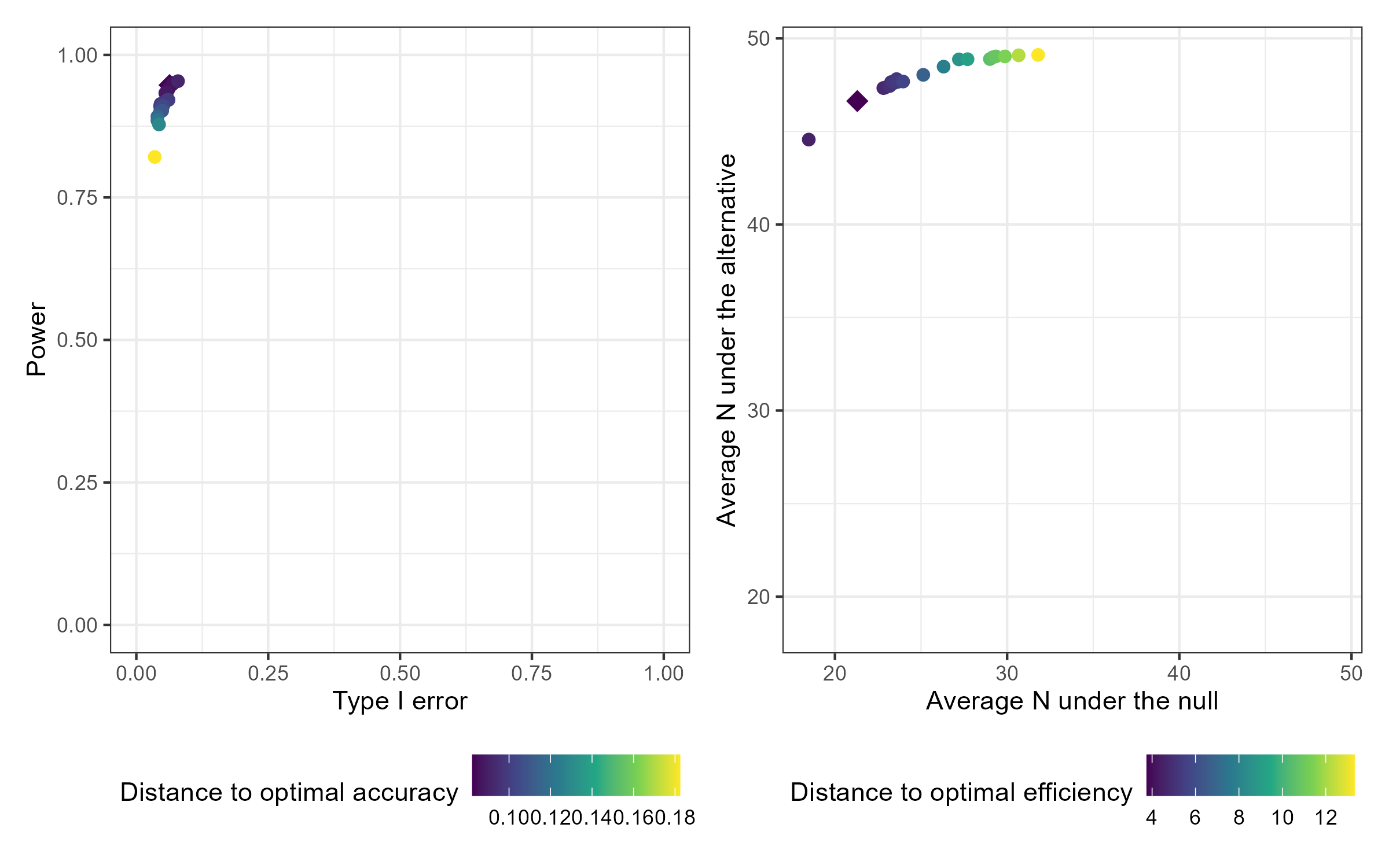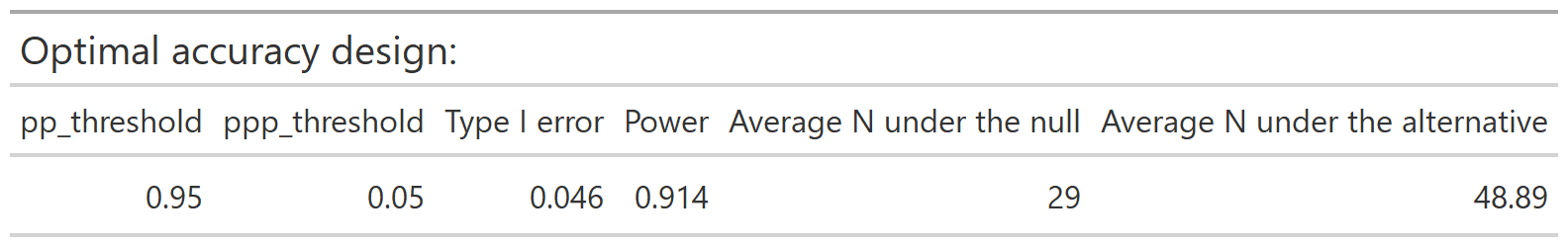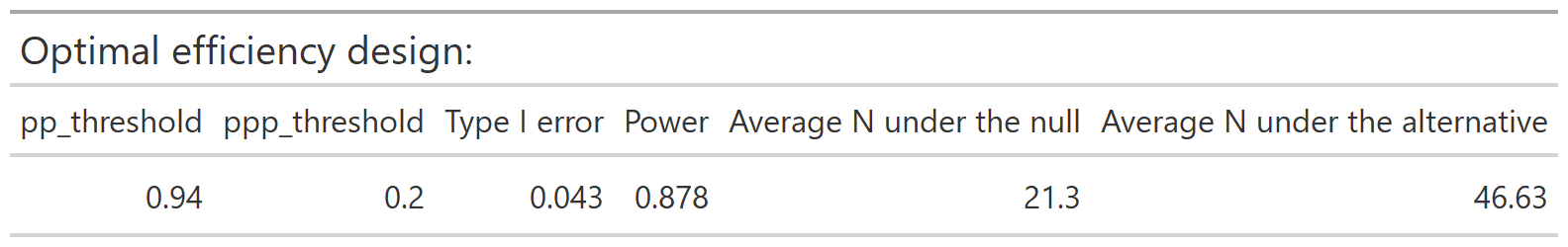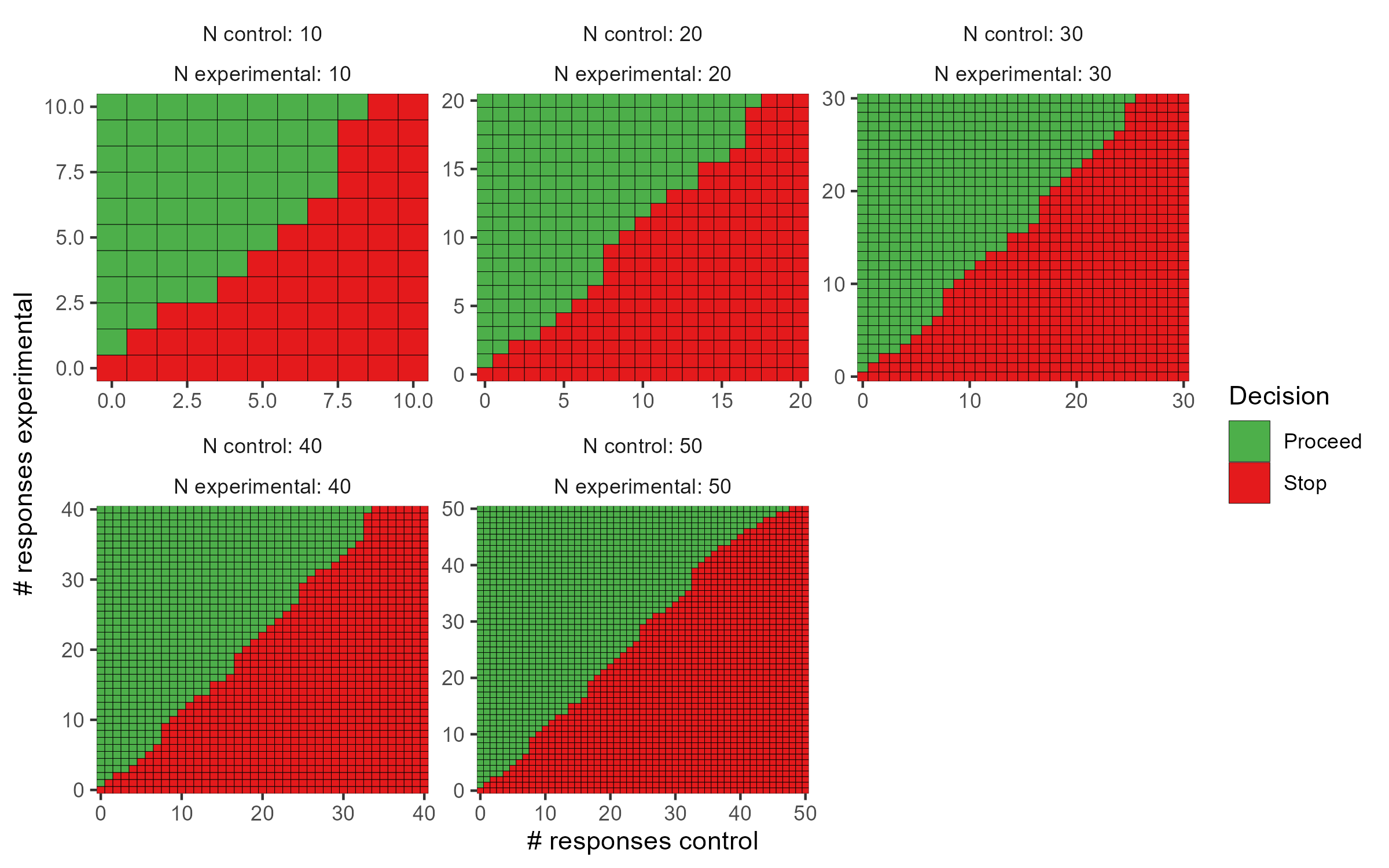## ppseq

The {ppseq} package provides functions to design clinical trials using Bayesian sequential predictive probability monitoring. Functionality is available to design one-arm or two-arm trials by searching over a grid of combinations of posterior and predictive thresholds and identifying the optimal design according to two criteria: accuracy and efficiency. Interactive plotting allows easy comparison of the various design options and easy trial implementation through decision rule plots.

## Installation

You can install the production version of ppseq from CRAN with:

``install.packages("ppseq")``

Or you can install the development version of ppseq from GitHub with:

``remotes::install_github("zabore/ppseq")``

## Basic usage

``library(ppseq)``

The primary function to search over a grid of combinations of posterior and predictive thresholds for a certain trial design is `calibrate_thresholds()`. This function is computationally intensive to varying degrees depending on the number of looks and the number of threshold combinations, and is best run on a server and/or with parallelization.

``````set.seed(12345)

calthresh <-
calibrate_thresholds(
p_null = c(0.2, 0.2),
p_alt = c(0.2, 0.5),
n = cbind(seq(10, 50, 10), seq(10, 50, 10)),
N = c(50, 50),
pp_threshold = seq(0.9, 0.95, 0.01),
ppp_threshold = seq(0.05, 0.2, 0.05),
delta = 0
)``````

The resulting design options can be compared interactively compared by passing the results to `plot()` with the option `plotly = TRUE`. Static versions of the plots are also available using the default option `plotly = FALSE`, which produces `ggplot` results. Plot output can optionally be filtered by desired range of type 1 error and minimum power. The default plots all design options. The results can also be viewed in tabular form by passing the results to `print()` with filtering options for the desired range of type 1 error and minimum power.

``plot(calthresh)``The optimal accuracy and optimal efficiency designs can be obtained by passing the results to the `optimize_design()` function, with filtering applied for the desired range of type 1 error and minimum power.

``optimize_design(calthresh, type1_range = c(0.025, 0.05), minimum_power = 0.8)``After selecting a design, we can obtain a set of decision rules to implement the trial, so that no calculations will be needed during the course of the trial. The `calc_decision_rules()` function will generate the decision rules to stop or continue at each interim look of the trial.

``````set.seed(123456)

opteffrules <-
calc_decision_rules(
n = cbind(seq(10, 50, 10), seq(10, 50, 10)),
N = c(50, 50),
theta = 0.94,
ppp = 0.2,
p0 = NULL,
delta = 0
)``````

The results can be displayed with interactive graphics by passing the results to `plot()` with the default option `plotly = TRUE`. Below are the static `ggplot` versions created with the option `plotly = FALSE` for demonstration purposes. Tabular results can be obtained by passing the results to `print()`.

``plot(opteffrules, plotly = FALSE)``See the vignettes for the one-sample and two-sample cases for additional details about available features and options.